# ISLR第五章Resampling Methods（重抽样方法）

2018/03/06 16:12

Resampling methods are an indispensable tool in modern statistics.

In this chapter, we discuss two of the most commonly used resampling methods, cross-validation and the bootstrap

For example,cross-validation can be used to estimate the test error associated with a given statistical learning method in order to evaluate its performance, or to select the appropriate level of flexibility.

The bootstrap is used in several contexts, most commonly model to provide a measure of accuracy of a parameter estimate or of a given selection statistical learning method.

5.1 Cross-Validation

In this section, we instead consider a class of methods that estimate the test error rate by holding out a subset of the training observations from thefitting process, and then applying the statistical learning method to those held out observations.

5.1.1The Validation Set Approach

Suppose that we would like to estimate the test error associated with fitting a particular statistical learning method on a set of observations. The validation set approach, displayed in Figure 5.1, is a very simple strategy validation for this task.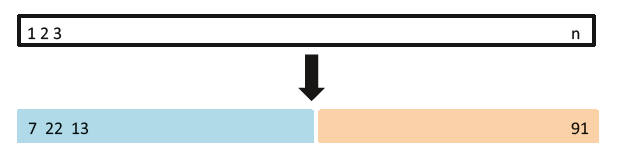FIGURE 5.1. A schematic display of the validation set approach. A set of n observations are randomly

split into a training set (shown in blue, containing observations 7, 22, and 13, among others) and a

validation set (shown in beige, and containing observation 91, among others). The statistical learning

method is fit on the training set, and its performance is evaluated on the validation set

1. 测试错误率的验证法估计的波动很大，这取决于具体哪些观测被包括在训练集中，哪些观测被包括在验证集中。
2. 在验证法中，只有一部分观测，验证集错误率可能会高估在整个数据集上拟合模型所得到的测试错误率。

5.1.2 Leave-One-Out Cross-Validation（留一交叉验证法LOOCV）

LOOCV也将观测集分为两类，但只留下一个单独的观测值(x1, y1)作为验证集，剩下的观测{(x2, y2), . . . , (xn, yn)}作为训练集

The LOOCV estimate for the test MSE is the average of these n test error estimates:LOOCV的原理如下图所示：FIGURE 5.3. A schematic display of LOOCV

1. 偏差更小，LOOCV 方法比验证集方法更不容易高估测试错误率
2. 第二，由于训练集和验证集分割的随机性，反复运用时会产生不同的结果，多次运用LOOCV 方法总会得到相同的结果:这是因为LOOCV 方法在训练集和验证集的分割上不存在随机性。

5.1.3 k-Fold Cross-Validation（k折交叉验证）

This approach involves randomly k-fold CV dividing the set of observations into k groups, or folds, of approximately equal size. The first fold is treated as a validation set, and the method is fit on the remaining k − 1 folds. The mean squared error, MSE1, is then computed on the observations in the held-out fold. This procedure is repeated k times; each time, a different group of observations is treated as a validation set. This process results in k estimates of the test error, MSE1,MSE2, . . . ,MSEk. The k-fold CV estimate is computed by averaging these values,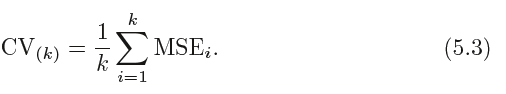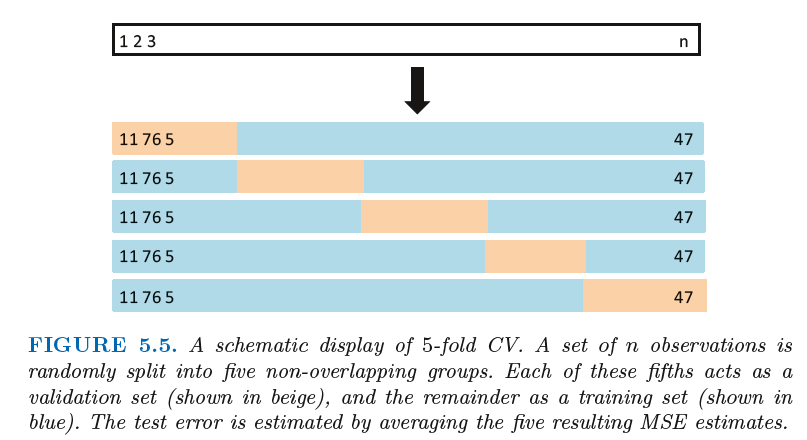5.1.4 Bias-Variance Trade-Off for k-Fold Cross-Validation

通常来说，考虑到上述因素，使用k 折交叉时一般令k=5 或k =10。因为从经验上来说，这些值使得测试错误率的估计不会有过大的偏差或方差。

5.1.5 Cross-Validation on Classification Problems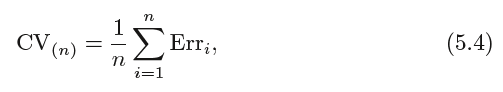where Erri = I(yi ！= ˆyi). The k-fold CV error rate and validation set error rates are defined analogously.

5.2 The Bootstrap

The bootstrap is a widely applicable and extremely powerful statistical tool bootstrap that can be used to quantify the uncertainty associated with a given estimator

or statistical learning method.

Suppose that we wish to invest a fixed sum of money in two financial assets that yield returns of X and Y , respectively, where X and Y are random quantities.

We will invest a fraction α of our money in X, and will invest the remaining 1 − α in Y . Since there is variability associated with the returns on these two assets,

we wish to choose α to minimize the total risk, or variance, of our investment. In other words, we want to minimize Var(αX +(1 −α)Y ). One can show that the

value that minimizes the risk is given by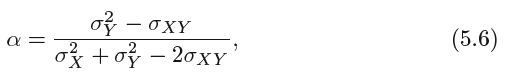where σ2X= Var(X), σ2Y= Var(Y ), and σXY = Cov(X, Y ).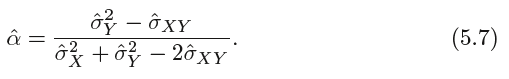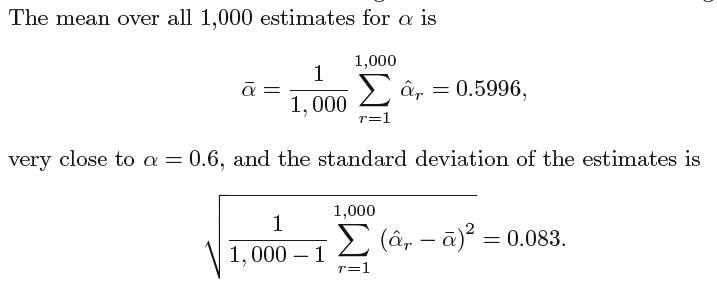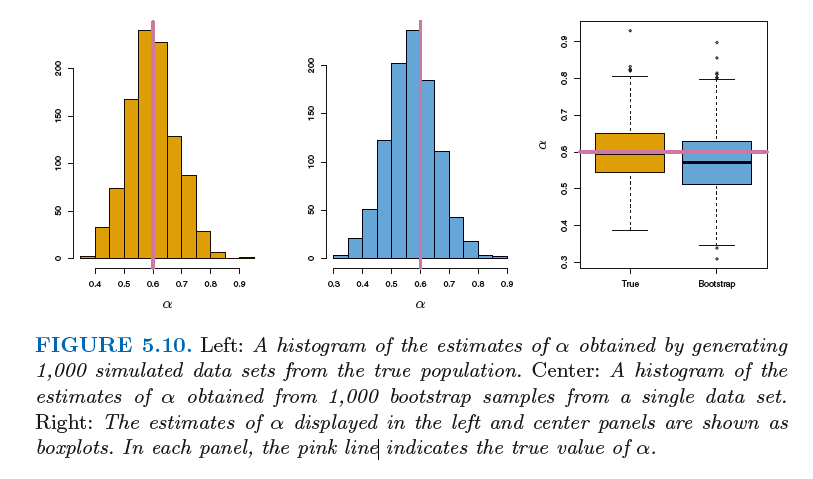However, the bootstrap approach allows us to use a computer to emulate the process of obtaining new sample sets，

so that we can estimate the variability of ˆα without generating additional samples. Rather than repeatedly obtaining

independent data sets from the population, we instead obtain distinct data sets by repeatedly sampling observations from the original data set.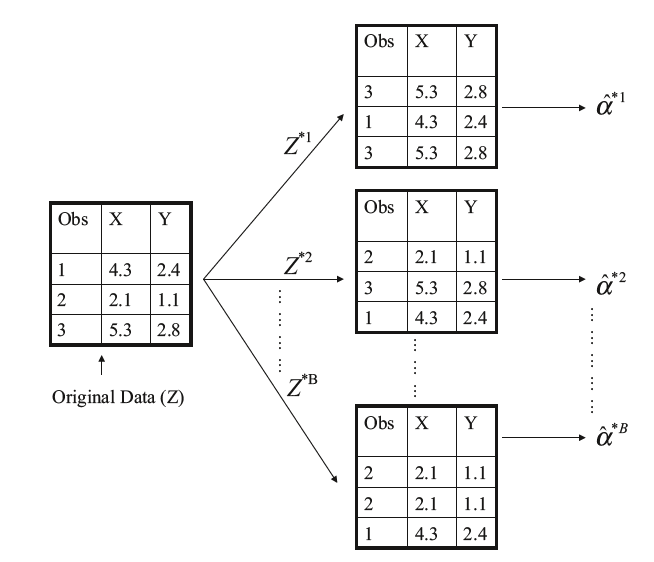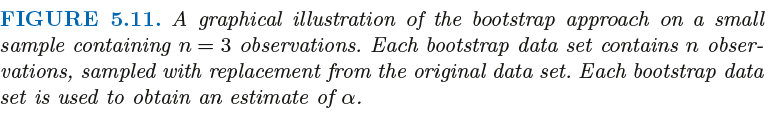### 作者的其它热门文章

0
0 收藏

0 评论
0 收藏
0